Learning ObjectivesBy the end of this class, you should know...What is an AVL Tree?What is the definition of a balanced tree according to the AVL tree property?How do AVL trees maintain their balance?What is the pseudocode for AVL insert?AVL TreesAn AVL tree (Georgy Adelson-Velsky and Evgenii Landis tree) is a self-balancing BST.AVL Tree PropertyRequires that the heights of the left and right children of every node differ by at most one.|left.height - right.height| <= 1Maintains a balanced tree to achieve O(log n) time for ALL operations.Review: What is the Big-O runtime of BST operations in best, worst and average cases?AVL Tree InsertWe need to insert nodes in such a way that the AVL tree property is satisfied.Thus, inside of our BST Nodes we are going to keep track of the height of the nodes.struct Node{    bstdata data;    int height;    Node* left;    Node* right;    Node(): left(NULL), right(NULL), height(0){}    Node(bstdata newdata): left(NULL), right(NULL), data(newdata), height(0){}};Insertion has 2 steps:Call BST insertRebalance the TreeTo balance the tree we use what are called "rotations" to alter the location of certain nodes.AVL Insert Pseudocode (Partial)Node avlInsert(Node root, int value)    //BST style insertionif (root == NULL)    return new Node(value)else if(root.data >= value)    root->left = avlInsert(root->left, value)else    root->right = avlInsert(root->right, value)//value has now been inserted//Is the resulting tree unbalanced?int balance = root->left->height - root->right->heightif (balance > 1 || balance < -1)    //re-balance the tree using rotations    //to be filled in later in classAVL Trees Maintain Balance by Readjusting the Tree -- i.e. doing what are called "Rotations" -- upon insertion and deletion.Rotations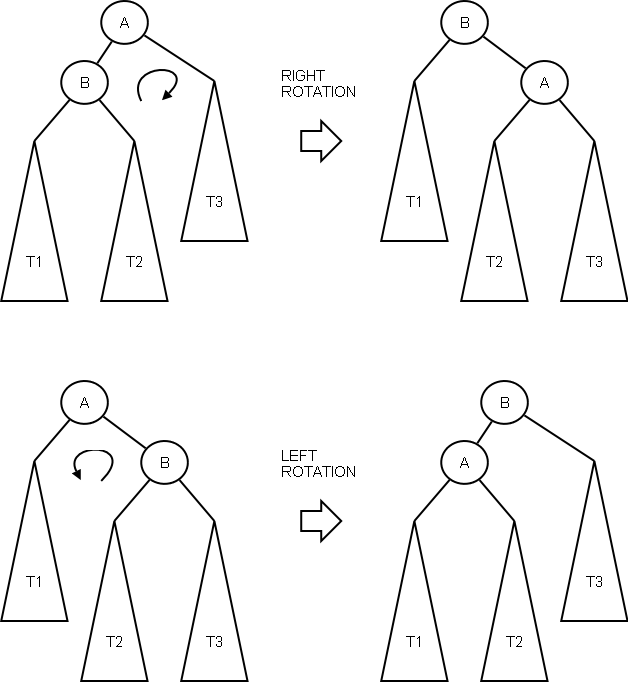With rotations, we want the height of the tree to become shorter (tree looks less like a linked list)Important: These rotations maintain the order of the BST: T1 < A < T2 < B < T3.Simple ExamplesImage source.Right Rotation        Before Rotation        After RotationNote that the upper case letters represent nodes in the tree, while the lower case letters represent possible subtrees.What happened to subtree c during the rotation?Example Right Rotation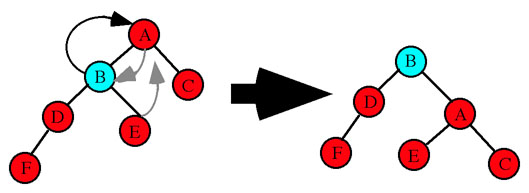Image source.Right Rotation PseudocodeNodeptr Right-Rotate(Nodeptr root)Nodeptr newRoot = root->leftroot->left = newRoot->rightnewRoot->right = rootroot->height = maxHeight(root->left, root->right) + 1newRoot->height = maxHeight(newRoot->left, newRoot->right) + 1return newRoot Left Rotation                      Before Left Rotation                               After Left RotationExample Left Rotation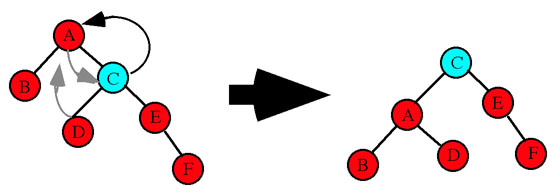Image source.Double RotationsSometimes a single rotation is not enough to balance a tree.Consider the following example:Our reaction might be to right rotate it, like so:However, we are now no better off than when we started.In cases like these (zig-zag pattern), we need to use a double rotation.We will need to do either a left-right rotation or a right-left rotation, depending on the situation.If the left subree is "right heavy" (i.e. left.height - right.height < -1)rotate left then rotate rightIf the right subtree is "left heavy" (i.e. left.height - right.height > 1)rotate right then rotate leftIn the example above, we have a left heavy right subtree.Therefore, we will do a left-right rotation.Step 1: Rotate left around the root.left, i.e. node A (to get a familar shape)Step 2: Rotate right around the root, i.e. B (to get a balanced tree)Example of left-right rotation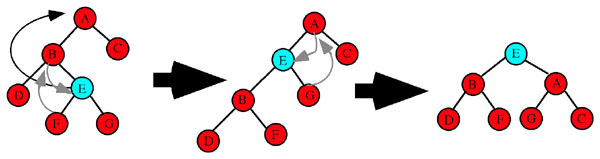Image source.If we have the opposite situation, where the right subtree is left heavy:Then, we must perform a right-left rotation.Step 1: Rotate right around root.right, i.e. A (to get a familar shape)Step 2: Rotate left around root, i.e. B (to get a balanced tree)Example of right-left rotation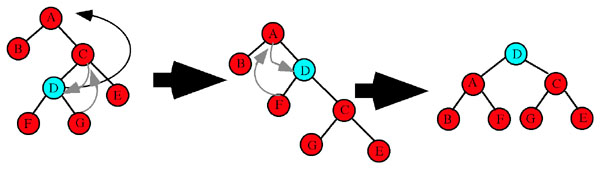Image source.For More Information: Double Rotation VideosPart 1Part 2Part 3AVL Insert Pseudocode (Full)Nodeptr avlInsert(Nodeptr root, int value){    //BST style insertion    if (root == NULL)        return(newNode(value));     if (value < root.data)        root->left  = avlInsert(root->left, value);    else        root->right = avlInsert(root->right, value);     //Check the balance    int balance = height(root->left) - height(root->right);    //Check for 4 cases     // Simple Right Rotation    if (balance > 1 && value < root->left->data)        return rightRotate(root);     // Simple Left Rotation    else if (balance < -1 && value > root->right->data)        return leftRotate(root);     // Left Right Case Double Rotation    else if (balance > 1 && value > root->left->value)        root->left =  leftRotate(root->left);        return rightRotate(root);     // Right Left Case Double Rotation    else if (balance < -1 && value < root->right->data)        root->right = rightRotate(root->right);        return leftRotate(root);       //return the node pointer    return root;For More Information: Video